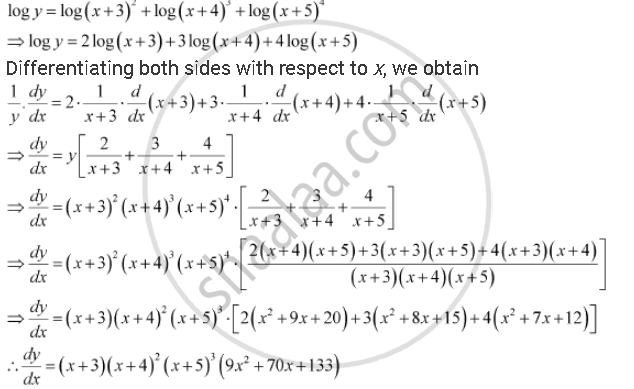# Differentiate the Function with Respect To X. (X + 3)2 . (X + 4)3 . (X + 5)4 - Mathematics

Differentiate the function with respect to x.

(x + 3)2 . (x + 4)3 . (x + 5)4

#### Solution

Let y = (x + 3)2 . (x + 4)3 . (x + 5)4

Taking logarithm on both the sides, we obtainConcept: Logarithmic Differentiation
Is there an error in this question or solution?

#### APPEARS IN

NCERT Class 12 Maths
Chapter 5 Continuity and Differentiability
Q 5 | Page 178